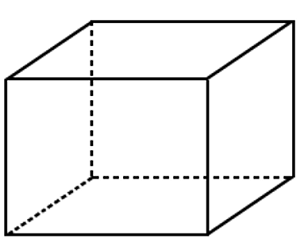# Data Cube Technology in Data Mining

In this tutorial, we will learn about the data cube technology in data mining, what is data cube technology used for, data cubes classifications. By Palkesh Jain Last updated : April 17, 2023

## What is Data Cube Technology?

A data cube is a three-dimensional (3D) (or higher) set of values that are typically used to describe the time series of data from an image. It is an abstraction of data to analyze aggregated information from a number of points of view. As a spectrally-resolved picture is interpreted as a 3-D volume, it is often useful for imaging spectroscopy.

The multidimensional extensions of two-dimensional tables may also represent a data cube. It can be viewed as a group of 2-D tables stacked on each other that are similar. Data cubes are used to represent data that is too abstract for a table of columns and rows to explain. As data in multidimensional matrices called Data Cubes is clustered or mixed. There are a few alternate names or alternatives to the data cube system, such as "Multidimensional databases," "materialized views," and "OLAP (On-Line Analytical Processing)."The general principle of this technique is to introduce those cost estimates that are commonly demanded. A data cube is a multi-dimensional ("n-D") sequence of values in computer programming contexts. The term data cube is usually used in contexts where these arrays are massively bigger than the main memory of the hosting computer; examples include multi-terabyte/petabyte data warehouses and image data time series.

From a subset of attributes in the database, a data cube is generated. To quantify attributes, unique attributes are selected, i.e. attributes whose qualities are of importance. The other attributes are chosen as usable attributes or measurements. The characteristics of the measurements are aggregated according to the proportions. For instance, XYZ can create a sales data warehouse to preserve records of the sales of the store for the time, object, branch, and location dimensions. These dimensions allow to store and keep track of details such as monthly transactions and the branches and areas where the goods were sold. Each dimension may be defined by a table, referred to as a dimensional table, which determines the dimensions. For eg, the item name, brand, and type attributes can include a dimension table for products.The data cube technique, with many implementations, is a fascinating method. In several examples, data cubes may be sparse and not every cell in each dimension would have matching data in the database.

## What is Data Cube Technology used for?

A multi-dimensional architecture is a data cube. The data cube is an abstract of data for displaying aggregated data from a variety of viewpoints. As the 'measure' attribute, the dimensions are aggregated, as the remaining dimensions are known as the 'function' attributes. In a multidimensional way, data is viewed on a cube. It is possible to display the aggregated and summarised facts with variables or attributes. This is the specification where OLAP plays a role.

For simple data analysis, data cubes are widely used. It is used to represent data as such quantities of company needs along with dimensions. Each cube dimension reflects some of the database's characteristics, such as revenue every day, month, or year.

## Data Cube Classifications

Data cubes are specifically grouped into two classifications. These are described below -

### 1. Multidimensional Data Cube

Centered on a structure where the cube is patterned as a multidimensional array, most OLAP products are created. Compared to other methods, these multidimensional OLAP (MOLAP) products typically provide a better performance, primarily because they can be indexed directly into the data cube structure to capture data subsets. The cube gets sparser as the number of dimensions is larger. That ensures that no aggregated data would be stored in multiple cells that represent unique attribute combinations. This in turn raises the storage needs, which can at times exceed undesirable thresholds, rendering the MOLAP solution untenable for massive, multi-dimensional data sets. Compression strategies may help, but their use may damage MOLAP's natural indexing.

### 2. Relational OLAP

The relational database architecture is used by Relational OLAP. Compared to a multidimensional array, the ROLAP data cube is used as a series of relational tables (approximately twice as many as the number of dimensions). Each of these columns, referred to as a cuboid, denotes a particular perspective.